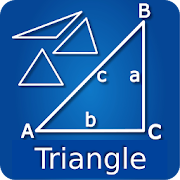# Triangle and Right Angle Calc - Lumos Educational App Store4.21
Price -fREE
\$0

#### DESCRIPTION:

Ipar, Triangle and Right Angle Free helps to solve math problems related to the Right Triangle (Pythagorean theorem, the trigonometric functions), computes the hypotenuse and all triangles. Useful for all types of triangles.. Right triangle. Only provides a data pair or three and press the = button You will get the value of other variables You'll get the process to obtain the solution, step by step solutions - Friendly and easy to use interface Just tap the name of a variable and see the process. On the right you have a menu with some user-friendly options (color, size number

#### OVERVIEW:

Triangle and Right Angle Calc is a free educational mobile app By .It helps students in grades HS practice the following standards HSF.TF.C.8.

This page not only allows students and teachers download Triangle and Right Angle Calc but also find engaging Sample Questions, Videos, Pins, Worksheets, Books related to the following topics.

1. HSF.TF.C.8 : Prove the Pythagorean identity sin2(θ) + cos2(θ) = 1 and use it to find sin(θ), cos(θ), or tan(θ) given sin(θ), cos(θ), or tan(θ) and the quadrant of the angle..

HS

#### STANDARDS:

HSF.TF.C.8

Developer:

Software Version: 11

Category:

### RELATED APPSEdSearch WebSearch